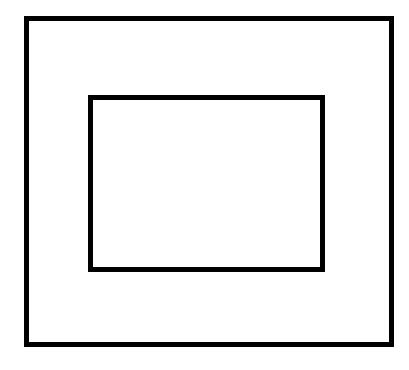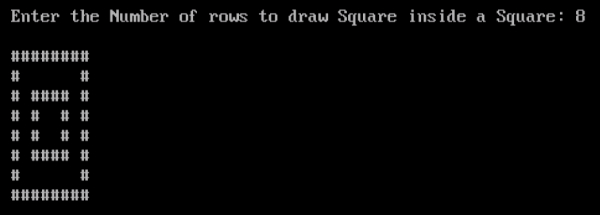# Program to print Square inside a Square in C

## Program Description

Print Square inside a Square as shown below## Algorithm

Accept the number of rows the outer Square to be drawn
Display the Outer Square with the number of rows specified by the User.
Display another square inside the outer square.

## Example

/* Program to print Square inside Square */
#include <stdio.h>
int main()
{
int r, c, rows;
clrscr();
printf("Enter the Number of rows to draw Square inside a Square: ");
scanf("%d", &rows);
printf("");
for (r = 1; r <= rows; r++){
for (c = 1; c <= rows; c++){
if ((r == 1 || r == rows || c == 1 || c == rows) || (r >= 3 && r <= rows - 2 && c >= 3 && c             <= rows - 2) && (r == 3 || r == rows - 2 || c == 3 || c == rows - 2)){
printf("#");
}
else{
printf(" ");
}
}
printf("");
}
getch();
return 0;
}

## Output